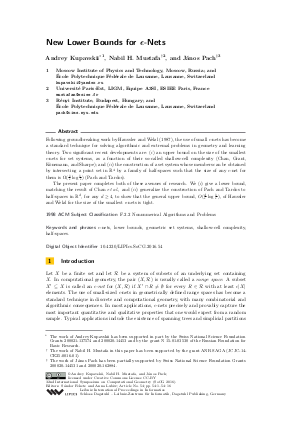Document# New Lower Bounds for epsilon-Nets

### Authors Andrey Kupavskii, Nabil Mustafa, János Pach## File

LIPIcs.SoCG.2016.54.pdf
• Filesize: 0.52 MB
• 16 pages

## Cite As

Andrey Kupavskii, Nabil Mustafa, and János Pach. New Lower Bounds for epsilon-Nets. In 32nd International Symposium on Computational Geometry (SoCG 2016). Leibniz International Proceedings in Informatics (LIPIcs), Volume 51, pp. 54:1-54:16, Schloss Dagstuhl - Leibniz-Zentrum für Informatik (2016)
https://doi.org/10.4230/LIPIcs.SoCG.2016.54

## Abstract

Following groundbreaking work by Haussler and Welzl (1987), the use of small epsilon-nets has become a standard technique for solving algorithmic and extremal problems in geometry and learning theory. Two significant recent developments are: (i) an upper bound on the size of the smallest epsilon-nets for set systems, as a function of their so-called shallow-cell complexity (Chan, Grant, Konemann, and Sharpe); and (ii) the construction of a set system whose members can be obtained by intersecting a point set in R^4 by a family of half-spaces such that the size of any epsilon-net for them is at least (1/(9*epsilon)) log (1/epsilon) (Pach and Tardos). The present paper completes both of these avenues of research. We (i) give a lower bound, matching the result of Chan et al., and (ii) generalize the construction of Pach and Tardos to half-spaces in R^d, for any d >= 4, to show that the general upper bound of Haussler and Welzl for the size of the smallest epsilon-nets is tight.
##### Keywords
• epsilon-nets; lower bounds; geometric set systems; shallow-cell complexity; half-spaces

## Metrics

• Access Statistics
• Total Accesses (updated on a weekly basis)
0

## References

1. P. K. Agarwal, J. Pach, and M. Sharir. State of the union (of geometric objects): A review. In J. Goodman, J. Pach, and R. Pollack, editors, Computational Geometry: Twenty Years Later, pages 9-48. American Mathematical Society, 2008.2. N. Alon. A non-linear lower bound for planar epsilon-nets. Discrete & Computational Geometry, 47(2):235-244, 2012.3. B. Aronov, E. Ezra, and M. Sharir. Small-size ε-nets for axis-parallel rectangles and boxes. SIAM J. Comput., 39(7):3248-3282, 2010.4. P. Ashok, U. Azmi, and S. Govindarajan. Small strong epsilon nets. Comput. Geom., 47(9):899-909, 2014.5. N. Bus, S. Garg, N. H. Mustafa, and S. Ray. Tighter estimates for epsilon-nets for disks. Comput. Geom., 53:27-35, 2016.6. T. M. Chan, E. Grant, J. Könemann, and M. Sharpe. Weighted capacitated, priority, and geometric set cover via improved quasi-uniform sampling. In Proceedings of Symposium on Discrete Algorithms (SODA), 2012.7. B. Chazelle and J. Friedman. A deterministic view of random sampling and its use in geometry. Combinatorica, 10(3):229-249, 1990.8. K. Clarkson and K. Varadarajan. Improved approximation algorithms for geometric set cover. Discrete &Computational Geometry, 37:43-58, 2007.9. K. L. Clarkson and P. W. Shor. Application of random sampling in computational geometry, II. Discrete & Computational Geometry, 4:387-421, 1989.10. D. Haussler and E. Welzl. Epsilon-nets and simplex range queries. Discrete &Computational Geometry, 2:127-151, 1987.11. J. Komlós, J. Pach, and G. J. Woeginger. Almost tight bounds for epsilon-nets. Discrete & Computational Geometry, 7:163-173, 1992.12. J. Matoušek. On constants for cuttings in the plane. Discrete & Computational Geometry, 20(4):427-448, 1998.13. J. Matoušek. Lectures in Discrete Geometry. Springer-Verlag, New York, NY, 2002.14. J. Matoušek, R. Seidel, and E. Welzl. How to net a lot with little: Small epsilon-nets for disks and halfspaces. In Proceedings of Symposium on Computational Geometry, pages 16-22, 1990.15. N. H. Mustafa, K. Dutta, and A. Ghosh. A simple proof of optimal epsilon-nets. Submitted, 2016.16. N. H. Mustafa and S. Ray. Near-optimal generalisations of a theorem of Macbeath. In Proceedings of the Symposium on Theoretical Aspects of Computer Science (STACS), pages 578-589, 2014.17. N. H. Mustafa and K. Varadarajan. Epsilon-approximations and epsilon-nets. In J. E. Goodman, J. O'Rourke, and C. D. Tóth, editors, Handbook of Discrete and Computational Geometry. CRC Press LLC, 2016, to appear.18. J. Pach and P. K. Agarwal. Combinatorial Geometry. John Wiley &Sons, New York, NY, 1995.19. J. Pach and G. Tardos. Tight lower bounds for the size of epsilon-nets. Journal of the AMS, 26:645-658, 2013.20. E. Pyrga and S. Ray. New existence proofs for epsilon-nets. In Proceedings of the Symposium on Computational Geometry (SoCG), pages 199-207, 2008.21. N. Sauer. On the density of families of sets. Journal of Combinatorial Theory, Series A, 13:145–147, 1972.22. Micha Sharir. On k-sets in arrangement of curves and surfaces. Discrete & Computational Geometry, 6:593-613, 1991.23. S. Shelah. A combinatorial problem; stability and order for models and theories in infinitary languages. Pacific Journal of Mathematics, 41:247–261, 1972.24. V. N. Vapnik and A. Ya. Chervonenkis. On the uniform convergence of relative frequencies of events to their probabilities. Theory of Probability and its Applications, 16(2):264-280, 1971.25. K. Varadarajan. Epsilon nets and union complexity. In Proceedings of the Symposium on Computational Geometry (SoCG), pages 11-16, 2009.26. K. Varadarajan. Weighted geometric set cover via quasi uniform sampling. In Proceedings of the Symposium on Theory of Computing (STOC), pages 641-648, New York, USA, 2010. ACM.X

Feedback for Dagstuhl Publishing